NEET  >  Pascal's Principle & Archimedes' Principle

# Pascal's Principle & Archimedes' Principle Notes | Study Physics Class 11 - NEET

## Document Description: Pascal's Principle & Archimedes' Principle for NEET 2022 is part of Physics Class 11 preparation. The notes and questions for Pascal's Principle & Archimedes' Principle have been prepared according to the NEET exam syllabus. Information about Pascal's Principle & Archimedes' Principle covers topics like and Pascal's Principle & Archimedes' Principle Example, for NEET 2022 Exam. Find important definitions, questions, notes, meanings, examples, exercises and tests below for Pascal's Principle & Archimedes' Principle.

Introduction of Pascal's Principle & Archimedes' Principle in English is available as part of our Physics Class 11 for NEET & Pascal's Principle & Archimedes' Principle in Hindi for Physics Class 11 course. Download more important topics related with notes, lectures and mock test series for NEET Exam by signing up for free. NEET: Pascal's Principle & Archimedes' Principle Notes | Study Physics Class 11 - NEET
 1 Crore+ students have signed up on EduRev. Have you?

3. Pascal's Principle

Some times while dealing with the problems of fluid it is desirable to know the pressure at one point is pressure at any other point in a fluid is known. For such types of calculations Pascal's Law is used extensively in dealing of static fluids. It is stated as

"The pressure applied at one point in an enclosed fluid is transmitted uniformly to every part of the fluid and to the walls of the container."

One more example can be considered better to explain the concept of Pascal's Principle. Consider the situation shown in figure, a tube having two different cross section S1 and S2, with pistons of same cross sections fitted at the two ends.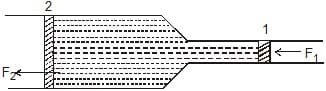If an external force F1 is applied to the piston 1, it creates a pressure p1 = F1/S1 on the liquid enclosed. As the whole liquid is at the same level, everywhere the pressure in the liquid is increased by p1. The force applied by the liquid on the piston 2 can be given as F2 =p2 × S2, and as the two pistons are at same level p2 = p1. Thus

F2 = p2 × S2

F2 =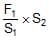....(2.21)

Equation (2.21) shows that by using such a system the force can be amplified by an amount equal to the ratio of the cross section of the two pistons. This is the principle of hydraulic press, we'll encounter in next few pages.

3.1 The Hydraulic Lift

Figure shows how Pascal's principle can be made the basis for a hydraulic lift. In operation, let an external force of magnitude F1 be exerted downward on the left input piston, whose area is S1. It result a force F2 which will act on piston 2 by the incompressible liquid in the device.

Here F2 = p2 × S2

And p2 = pB -rgh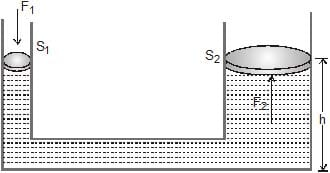Where pB is the pressure on the bottom of the device which can be given as :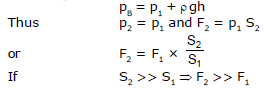4. Archimede's Principle

If a heavy object is immersed in water, it seems to weight less than when it is in air. This is because the water exerts an upward force called buoyant force. It is equal to the weight of the fluid displaced by the body.

A body wholly or partially submerged in a fluid is buoyed up by a force equal to the weight of the displaced fluid.

This result is known as Archimedes' principle.

Thus, the magnitude of buoyant force (F) is given by,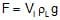Here, Vi = immersed volume of solid rL = density of liquid

and g = acceleration due to gravity

Note :  Point of Application of buoyant force is centre of liquied displaced

Proof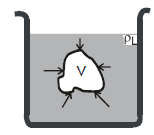Consider an arbitraily shaped body of volume V placed in a container filled with a fluid of density rL. The body is shown completely immersed, but complete immersion is not essential to the proof. To begin with, imagine the situation before the body was immersed. The region now occupied by the body was filled with fluid, whose weight was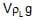. Because the fluid as a whole was in hydrostatic equilibrium, the net upwards force (due to difference in pressure at different depths) on the fluid in that region was equal to the weight of the fluid occuping that region.

Now, consider what happens when the body has displaced the fluid. The pressure at every point on the surface of the body is unchanged from the value at the same location when the body was not present. This is because the pressure at any point depends only on the depth of that point below the fluid surface. Hence, the net force exerted by the surrounding fluid on the body is exactly the same as the exerted on the region before the body was present. But we know the latter to be, the weight of the displaced fluid. Hence, this must also be the buoyant force exerted on the body. Archimedes' principle is thus, proved.

Ex.5 Beaker cicular cross-section of radius 4 cm is filled with mercury upto a height of 10 cm. Find the force exerted by the mercury on the bottom of the beaker. The atmopheric pressure = 105 N/m2. Density of mercury = 13600 kg/m3. Take g = 10 m/s2

Sol. The pressure at the surface = atmospheric pressure = 105 N/m2.

The pressure at the bottom = 105 N/m2 hrg

= 105 N/m2 + (0.1 m)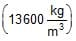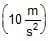= 105 N/m2 + 13600 N/m2 = 1.136 × 105 N/m2

The force exerted by the mercury on the bottom

= (1.136 × 105 N/m2) × (3.14 × 0.04 m × 004 m) = 571 N

Ex.6 A cubical block of iron 5 cm on each side is floating on mercury in a vessel.

(i) What is the height of the block above mercury level ?

(ii) What is poured in the vessel until it just covers the iron block. What is the height of water column.

Density of mercury = 13.6 gm/cm3

Density of iron 7.2 gm/cm3

Sol. Case-I : Suppose h be the height of cubical block of iron above mercury.

Volume of iron block = 5 ×5 × 5 = 125 cm3

Mass of iron block = 125 × 7.2 = 900 gm

Volume of mercury displaced by the block = 5 × 5 × (5 -h) cm3

Mass of mercury displaced = 5 × 5 (5 -h) × 13.6 gm

By the law floatation,

weight of mercury displaced = weight of iron block

5 × 5 (5 -h) × 13.6 = 900

or (5 -h) =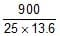= 2.65           ⇒ h = 5 -2.65 = 2.35 cm

Case - II : Suppose in this case height of iron block in water be x. The height of iron block in mercury will be (5 -x) cm.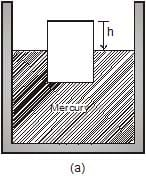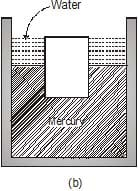Mass of the water displaced = 5 × 5 × (x) × 1

Mass of mercury displaced = 5 × 5 × (5 -x) × 13.6

So, weight of water displaced weight of mercury displaced = weight of iron block

or 5 × 5 × x × 1 5 × 5 × (5 -x) × 13.6 = 900

or x = (5 -x) × 13.6 = 36

x = 2.54 cm

Ex.7 A tank contianing water is placed on spring balanced. A stone of weight w is hung and lowered into the water without touching the sides and the bottom of the tank. Explain how the reading will change.

Sol. The situation is shown in figure.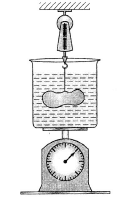Make free-body diagrams of the bodies separately and consider their equilibrium. Like all other forces, buoyancy is also exerted equally on the two bodies in contact. Hence it the water exerts a buoyant force, say, B on the stone upward, the stone exerts the same force on the water downward. The forces acting on the 'water container' system are : W, weight of the system downward, B, buoyant force of the stone downard, and the force R of the spring in the upward direction. For equilibrium

R = W + B

Thus the reading of the spring scale will increase by an amount equal to the weight of the liquid displaced, that is, by an amount equal to the buoyant force.

Ex.8 A cylindrical vessel containing a liquid is closed by a smooth piston of mass m as shown in figure.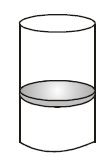The area of cross-section of the piston is A. If the atmopheric pressure is P0, find the pressure of the liquid just below the prism.

Sol. Let the pressure of the liquid just below the piston be P.

The forces acting on the piston are

(a) its weight, mg (downward)

(b) force due to the air above it, P0A(downward)

(c) force due to the liquid below it, PA (upward)

If the piston is in equilibrium

PA = P0A + mg or P = P0 +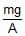Ex.9 A rubber ball of mass m and radius r is submerged in water to a depth h released. What height will the ball jump up to above the surface of the water ? Neglect the resistance of water and air.

Sol. Let the ball go up by x above the level of water.

Let us now consider energy conservation between the initial and final positions. In both the positions kinetic energy of the body is zero. The potential energy in the first position with reference to the water level is -mgh plus the work done by an external agent against the buoyant force which is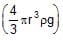h,

where r is the density of the water

or x-mghh = mgx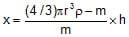Ex.10 A cube of wood supporting a 200 g mass just floats in water. When the mass is removed, the cube rises by 2 cm. What is the size of the cube ?

Sol. If, l = side of cube, h = height of cube above water and r = density of wood.

Mass of the cube = l3r

Volume of cube in water = l(l -h)

Volume of the displaced water =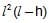As the tube is floating

The document Pascal's Principle & Archimedes' Principle Notes | Study Physics Class 11 - NEET is a part of the NEET Course Physics Class 11.
All you need of NEET at this link: NEET

## Physics Class 11

126 videos|449 docs|210 tests
 Use Code STAYHOME200 and get INR 200 additional OFF

## Physics Class 11

126 videos|449 docs|210 tests

### How to Prepare for NEET

Read our guide to prepare for NEET which is created by Toppers & the best Teachers

Track your progress, build streaks, highlight & save important lessons and more!

,

,

,

,

,

,

,

,

,

,

,

,

,

,

,

,

,

,

,

,

,

;